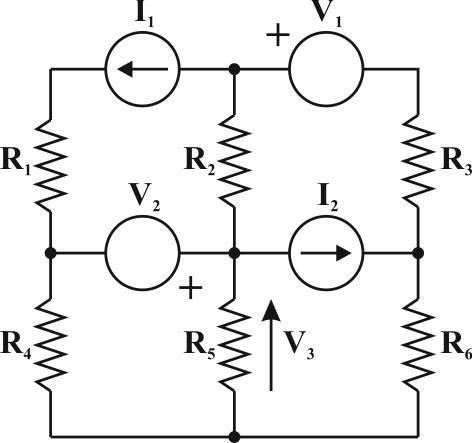# Resistive Network. - nodal analysis

VisualStudio

## Homework Statement

http://learn.lboro.ac.uk/file.php/1844/Quiz_figures/Chins_Files/Q11.jpg

In the above circuit

V1=17 Volts

V2=8.5 Volts

V3=4.25 Volts

I1= 4 Amps

I2= 5 Amps

R1=4.25 Ohms

R3=6.375 Ohms

R4=1.0625 Ohms

R5=2.125 Ohms

R6=2.125 Ohms

Calculate the following:

a) Magnitude of the current through R5:

b) The resistance R2:

a) Magnitude of the voltage across I1:

## The Attempt at a Solution

I am really unsure on how to start this question, any starting tips would be great please, this was a tutorial set in my college class.## Answers and Replies

Staff Emeritus
Have you looked at where the given voltage V3 is located?VisualStudio
what do you mean by that sorry?

Staff Emeritus
You're wanting guidance to answer part (a)?

VisualStudio
I think i know how to work out Part A - Im just guessing its Voltage divided by R?
Its part b and c, that i am stuck with

Staff Emeritus
I think i know how to work out Part A - Im just guessing its Voltage divided by R?
Guessing??

Its part b and c, that i am stuck with
Can you work out how many volts across R4?
Hence, determine the current through R4 .... and so on, working your way around the circuit until you have determined all parameters needed to answer the questions.

VisualStudio
Sorry I'm confused now. Would I need nodal analysis for this and convert all of these to current sources?

Mentor
You do not have to convert anything, and you can do it step by step. There is no need for multiple unknowns at the same time. NascentOxygen showed you the first two steps.

Staff Emeritus